High School Algebra - Quadratic Equations: Help and Review Chapter Exam

Exam Instructions:

Choose your answers to the questions and click 'Next' to see the next set of questions. You can skip questions if you would like and come back to them later with the yellow "Go To First Skipped Question" button. When you have completed the practice exam, a green submit button will appear. Click it to see your results. Good luck!

Answered 0 of 25

Page 1

Question 1 1. Which statement is true about the inequality below?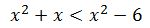Question 2 2. Find the roots of the equation.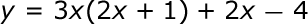Page 2

Question 6 6. Find the roots of the equation below.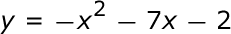Question 8 8. Which of the following are the solutions to the equation below?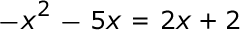Page 3

Question 11 11. Rewrite the quadratic below in standard form: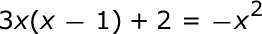Question 15 15. Solve this inequality.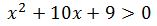Page 4

Question 16 16. What are the zeros in the following quadratic equation?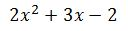Question 19 19. Use the quadratic formula to find the solutions to the equation below.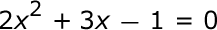Question 20 20. Solve the equation.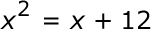Page 5

Question 23 23. What is the b-value of the quadratic equation below?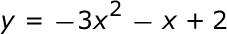Question 25 25. Solve the following inequality.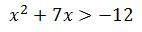High School Algebra - Quadratic Equations: Help and Review Chapter Exam Instructions

Choose your answers to the questions and click 'Next' to see the next set of questions. You can skip questions if you would like and come back to them later with the yellow "Go To First Skipped Question" button. When you have completed the practice exam, a green submit button will appear. Click it to see your results. Good luck!

Support